# Full-stack Quantum Hardware Design

Stefan Krastanov | UMass Amherst, MIT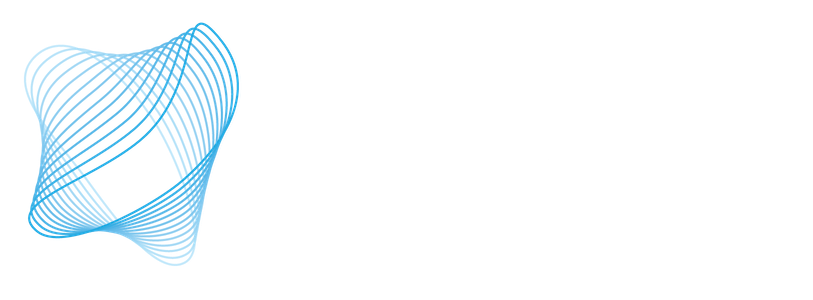U. Arizona, Harvard, MIT, Yale, BYU, U. Chicago, Howard University, U. Oregon, UMass Amherst, NAU

## Computing in the Real Universe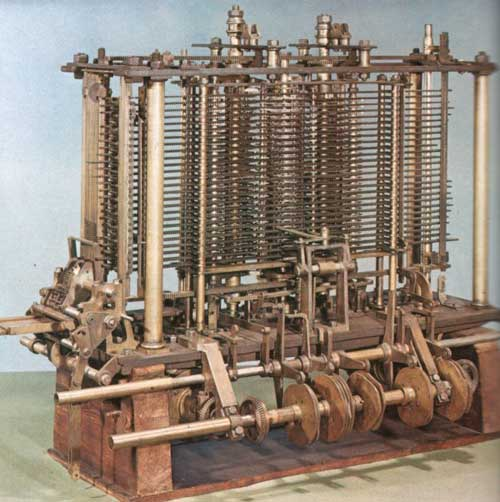The Analytical Engine, envisioned by Babbage and Lovelace as a programmable computer two centuries ago¹.

### Why care about a quantum model of computation?

BQP seems bigger than P or BPP.

Useful problems become easy on a quantum device.

Going in the other direction: theoretical computer science can inform theoretical physics.

### Where is the Quantum Advantage?

Computing a function on all possible inputs at the same time?

Consider searching for the zero $z$ of a function $\textrm{f}$
$\textrm{f}(z)=0$
$\textrm{f}(x)=1$ for $x\ne z$

$$\begin{matrix} x_1 \\ x_2 \\ z \end{matrix}$$

possible inputs

$$\begin{pmatrix} p_{x_1} \\ p_{x_2} \\ p_{z} \end{pmatrix}$$

initial state of the computer

$$\hat{M} \begin{pmatrix} p_{x_1} \\ p_{x_2} \\ p_{z} \end{pmatrix}$$

executing the program

$$\begin{pmatrix} 0 \\ 0 \\ 1 \end{pmatrix}$$

desired final state

$$\begin{matrix} x_1 \\ x_2 \\ z \end{matrix}$$

possible inputs

$$\begin{pmatrix} p_{x_1} \\ p_{x_2} \\ p_{z} \end{pmatrix}$$

initial state of the computer

$$\hat{M} \begin{pmatrix} p_{x_1} \\ p_{x_2} \\ p_{z} \end{pmatrix}$$

executing the program

$$\begin{pmatrix} 0 \\ 0 \\ 1 \end{pmatrix}$$

desired final state

$\hat{M}$ is "stochastic" in a probabilistic computer
$\hat{M}$ is "unitary" in a quantum computer

$$b \in \{0,1\}$$

classical bit

$$\begin{pmatrix}p_0\\p_1\end{pmatrix} \in \mathbb{R}^2$$ $$p_0+p_1=1$$

classical probabilistic bit

$$\begin{pmatrix}c_0\\c_1\end{pmatrix} \in \mathbb{C}^2$$ $$|c_0|^2+|c_1|^2=1$$

quantum bit (qubit)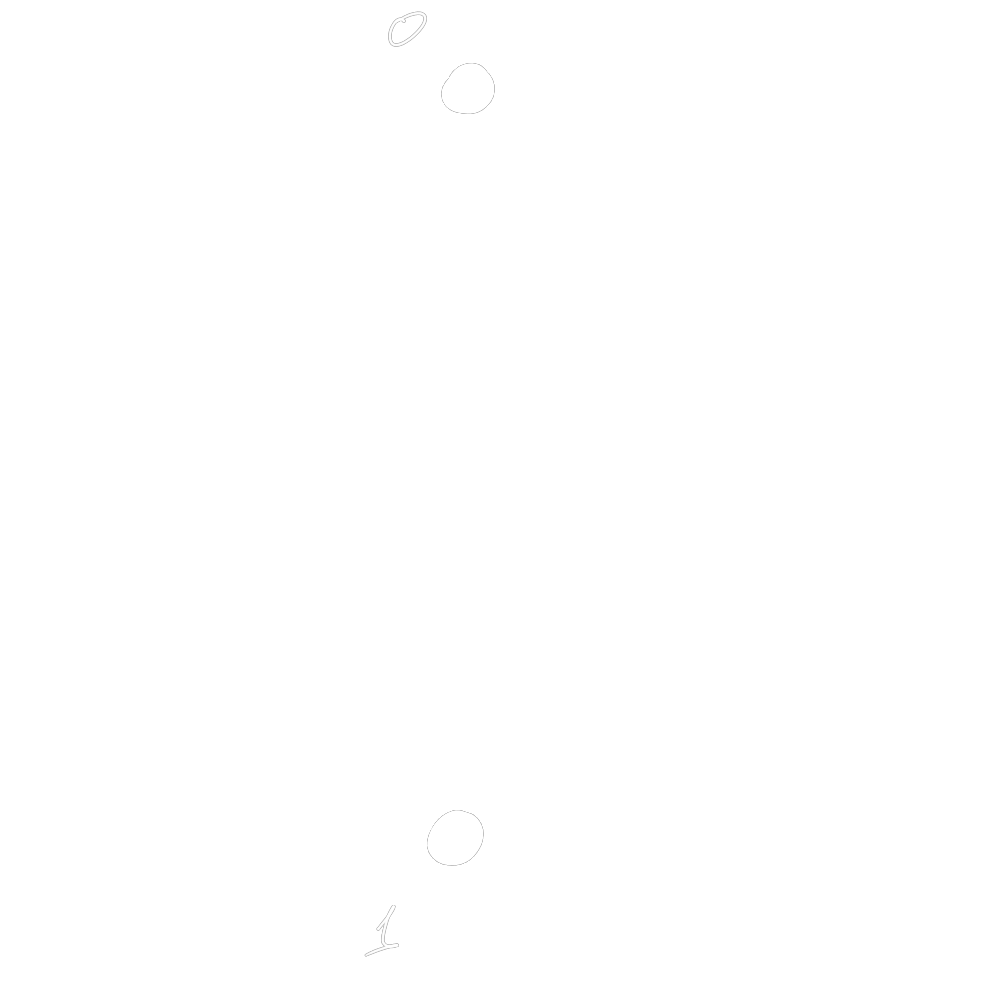classical bit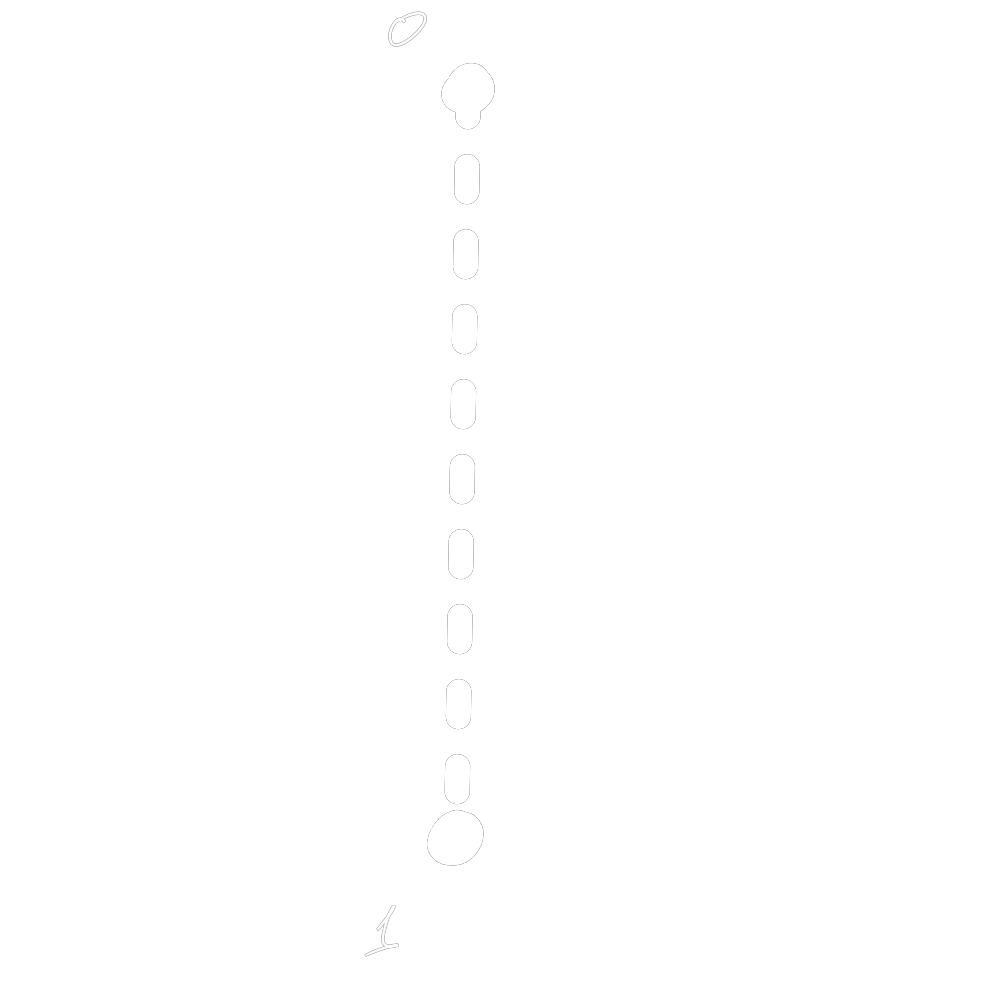classical probabilistic bit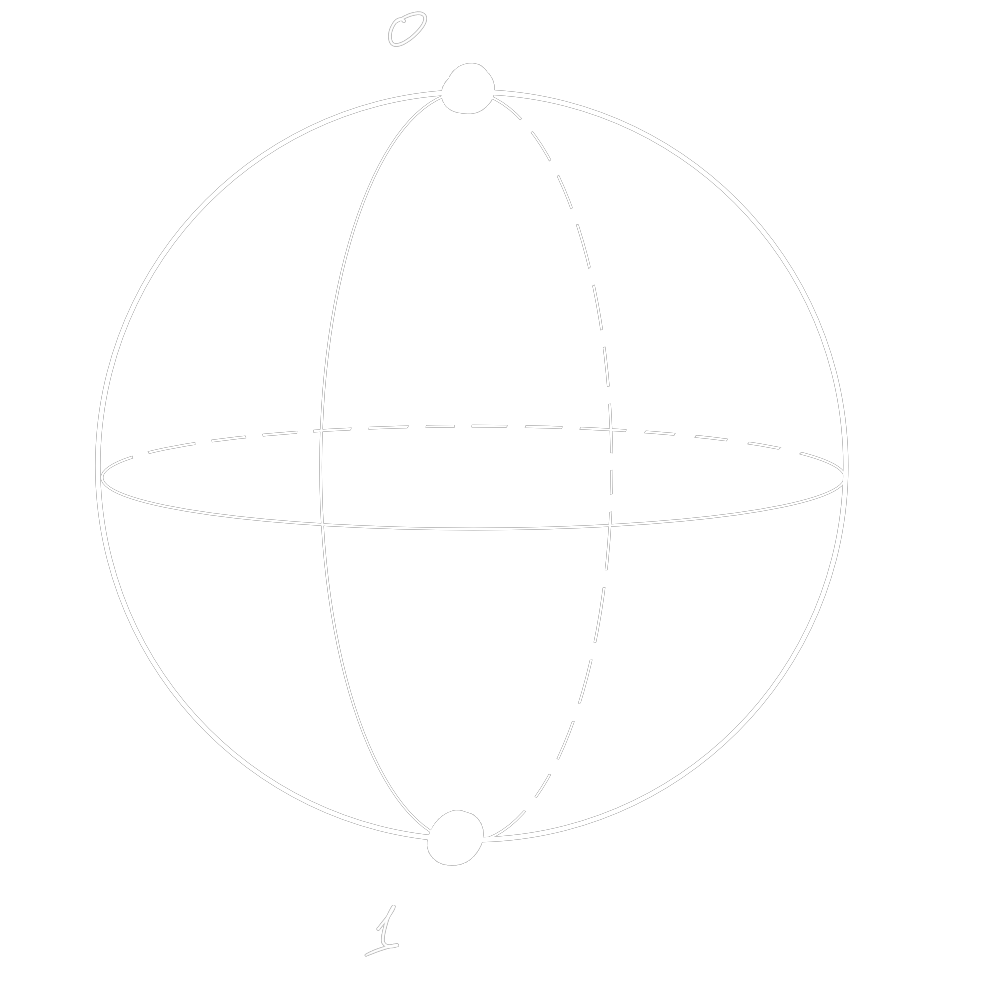quantum bit (qubit)

### Why is it taking so long?The Analytical Engine, envisioned by Babbage and Lovelace as a programmable computer two centuries ago¹.
1. von Neumann
The Synthesis of Reliable Organisms […]
More than a hundred years between the conception of programmable machines and their realization.

1. von Neumann
The Synthesis of Reliable Organisms […]

## The Quantum Technology Stack

### We have the fastest "algebra of quantum error correction" tool


a = random_pauli(1_000_000_000);
b = random_pauli(1_000_000_000);
@benchmark mul_left!(a,b)
# Time  (median):     32.246 ms

1 Giga qubit ops in 32ms thanks to SIMD.jl and Polyester.jl
1. Krastanov and contributors
QuantumClifford.jl

... and work on GPU acceleration for error correction codes (ECC)¹

... and a library of codes and ECC circuit compilers²

... and expander-graph LDPC code generators³

Possible thanks to CUDA.jl and Nemo.jl

1. past and current undergrad projects at MIT and Hampshire
2. past and current undergrad projects at GSoC and UMass
QuantumExpanders.jl

### And the occasional algorithmic discovery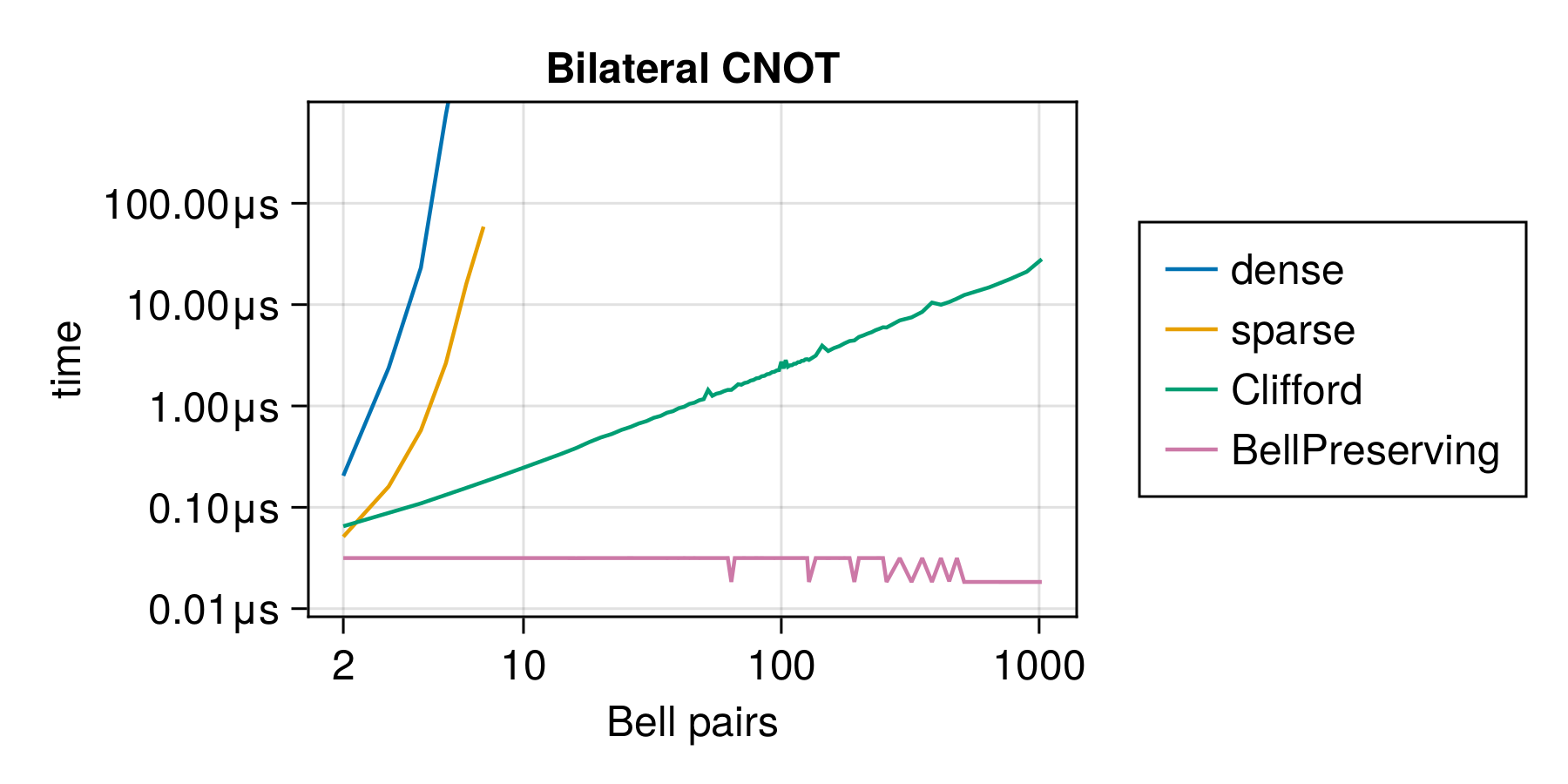Time to perform a pair of CNOT gates, depending on formalism
1. past undergrad projects at MIT (Shu Ge)
BPGates.jl

### The wider Julia ecosystem

QuantumOptics.jl, ITensors.jl, Yao.jl, quantum chemistry and solid state tools, EM tools, multiphisics classical tools, etc

## Full-Stack Design and Optimization Toolkit

### Symbolic description of quantum logic


julia> X1⊗L1
|+⟩|1⟩

julia> express(X1⊗L1)
Ket(...) # a dense vector from
# QuantumOptics.jl

julia> express(X1⊗L1, CliffordRepr())
X_    # an exponentially more-efficient
-_Z    # representation from
# QuantumClifford.jl

Build upon Symbolics.jl and many "backend" library.

traits = [Qubit(), Qubit(), Qumode()]
reg = Register(traits)


A register "stores" the states being simulated.

### Translation to many simulator backends


initialize!(reg, X₁)


A register's slot can be initialized to an arbitrary state, e.g. $|x_1\rangle$ an eigenstate of $\hat{\sigma}_x$.

### Translation to many simulator backends


initialize!(reg, X₁)
initialize!(reg, Z₁)
apply!((reg, reg), CNOT)


Arbitrary quantum gates or channels can be applied.

### Translation to many simulator backends


initialize!(reg, X₁)
initialize!(reg, Z₁)
apply!((reg, reg), CNOT)


Arbitrary quantum gates or channels can be applied.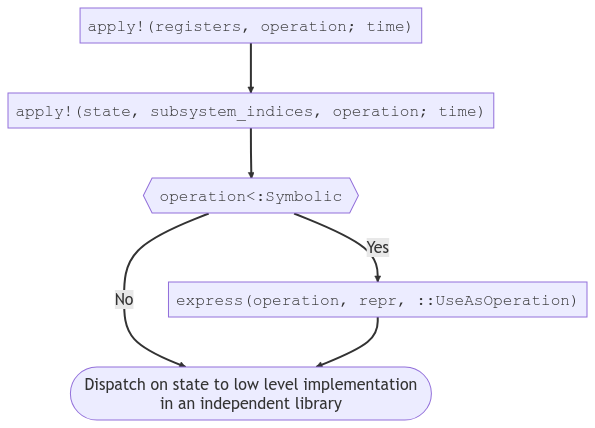### Discrete event scheduler

Locks and channels, message passing, delays, concurrency, agent-based sims...

### QuantumSavory.jl

github.com/Krastanov/QuantumSavory.jl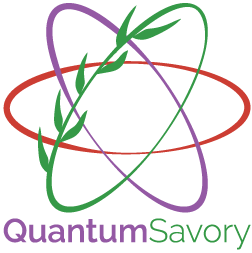prerelease...

We are hiring both at UMass Amherst and MIT:
software engineering
quantum science

Message at stefankr@mit.edu, skrastanov@umass.edu

Including work done by Vaishnavi Addala and Shu Ge, in coordination with Dirk Englund.

Consider gradschool or postdoc at UMass Amherst:Design of optical/mechanical/spin devices with Sandia, Mitre, and MIT.

Working on practical LDPC ECC in networking and computing with CQN.

Creating new tools for the entire community.